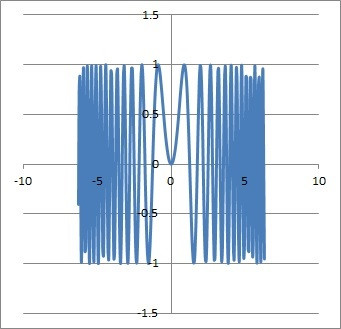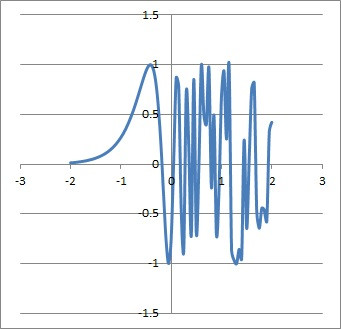# Function Composition (5 Common Questions Answered)

Function composition is often used in algebra and calculus, and it has many interesting properties.

So, what is function composition?  Function composition uses the output of a function as the input for another function. Function composition combines two functions into a new one. The notation f○g(x) means the function composition f(g(x)). Function composition is associative, but not always invertible, commutative, or distributive.

Of course, the range of the inner function has to be compatible with the domain of the outer function when we do function composition.

In this article, we’ll talk about function composition and answer some common questions. We’ll also look at some examples to show how it works.

Let’s get started.

## What Is Function Composition?

Function composition is a way of combining two functions by connecting their input/output.  It is different from addition, subtraction, multiplication, or division of functions.

Function composition uses the output of the inner function as the input for the outer function. In this way, function composition combines two functions into a new one.

We often use the notation f○g(x) to represent function composition with an outer function of f(x) and an inner function of g(x).

The notation f○g(x) is equivalent to f(g(x)).  If we switch the order of functions in the notation, we get g○f(x), which is equivalent to g(f(x)).

Note that we can compose a function with itself to get f○f(x), which is equivalent to f(f(x)).

We can also take the inverse of a function composition.

### What Is The Function Composition Inverse?

The inverse of the function composition f(g(x)) is

• g-1(f-1(x))

where

• f-1(x) is the inverse function of f(x)
• g-1(x) is the inverse function of g(x)

Note: this assumes that both f(x) and g(x) are invertible functions.

So f○g(x) = f(g(x)) and (f○g)-1(x) = g-1(f-1(x)).

We can verify the inverse of f○g(x) as follows:

• (f○g)((f○g)-1(x))
• =f○g(g-1(f-1(x)))
• =f(g(g-1(f-1(x))))
• =f(f-1(x))  [g(g-1(f-1(x))) = f-1(x), since g(x) and g-1(x) are inverses]
• =x  [since f(x) and f-1(x) are inverses

Similarly, we can show that:

• (f○g)-1(f○g(x))
• g-1(f-1(f○g(x)))
• g-1(f-1(f(g(x))))
• g-1(g(x)))  [f-1(f(g(x))) = g(x), since f(x) and f-1(x) are inverses]
• =x  [since g(x) and g-1(x) are inverses

For a composition of three functions, we would do the same “reverse” composition to find the inverse.  In other words, for the function composition f(g(h(x))), the inverse is h-1(g-1(f-1(x))).

### Is Function Composition Commutative?

Function composition is not commutative. There are certain cases where f○g(x) = g○f(x) (for example, when f(x) = x and g(x) = x), but this does not hold true in general.

Let’s look at a counterexample to show that function composition is not always commutative.

Let f(x) = x2 and g(x) = x + 1.

First, we will calculate the composition function f○g(x):

• f○g(x)
• =f(g(x))
• =f(x+1)
• =(x+1)2
• =x2 + 2x + 1

Now, we will calculate the composition function f○g(x):

• g○f(x)
• =g(f(x))
• =g(x2)
• =x2 + 1

Comparing the two compositions, we get:

• f○g(x) = x2 + 2x + 1
• g○f(x) = x2 + 1

These two functions are not equal (except when x = 0).  So, this proves that function composition is not commutative in general.

### Is Function Composition Distributive?

Function composition is not distributive. There are certain cases where f○(g + h)(x) = f○g(x) + f○h(x)  (for example, when f(x) = x, g(x) = x, and h(x) = x), but this does not hold true in general.

Let’s look at a counterexample to show that function composition is not always distributive.

Let f(x) = x2, g(x) = x, and h(x) = 1.

First, we will calculate the composition function f○(g+h)(x):

• f○(g+h)(x)
• =f(x + 1)
• =(x+1)2
• =x2 + 2x + 1

Now, we will calculate the sum f○g(x) + f○h(x):

• f○g(x) + f○h(x)
• =f(g(x)) + f(h(x))
• =f(x) + f(1)
• =x2 + 12
• =x2 + 1

Comparing the two results, we get:

• f○(g+h)(x) = x2 + 2x + 1
• f○g(x) + f○h(x)= x2 + 1

These two functions are not equal (except when x = 0).  So, this proves that function composition is not distributive in general.

### Is Function Composition Associative?

Function composition is associative.  This means that for functions f(x), g(x), and h(x):

• f○g(h(x)) = f(g○h(x))

The reason is that both sides comes out to f(g(h(x))).  We can also reason that both sides are the inverse of h-1(g-1(f-1(x))), and since the inverse of a function isunique, both sides of the equation must be the same function.

### Function Composition Derivative

The derivative of the function composition f○g(x) is given by:

• d/dx[f○g(x)] = f’(g(x))*g’(x)  [chain rule]

where f’(x) is the derivative of f(x) and g’(x) is the derivative of g(x).

We can apply the chain rule to as many “layers” of function composition as needed.  Here is what it looks like for a composition of three functions, f(g(h(x))):

• d/dx[f(g(h(x)))] = f’(g(h(x)))*g’(h(x))*h’(x)

### Function Composition Examples

Let’s take a look at some examples of function composition to see how they work (both with and without numerical inputs).

#### Example 1: Function Composition Of Two Functions

Let’s say we have the functions f(x) = sin(2x) and g(x) = x2.

We can find the function composition f○g(x) as follows:

• f○g(x)
• =f(g(x))
• =f(x2)
• =sin(2x2)

You can see the graph of this function composition below.Here we have the graph of the function composition f(g(x)) for f(x) = sin(2x) and g(x) = x2, which gives us the function sin(2x2).

#### Example 2: Function Composition Of Three Functions

Let’s say we have the functions f(x) = sin(2x), g(x) = ex, and h(x) = 3x + 1.

We can find the function composition f(g(h(x))) as follows:

• f(g(h(x)))
• =f(g(3x+1))
• =f(e3x+1)
• =sin(2e3x+1)

You can see the graph of this function composition below.Here we have the graph of the function composition f(g(h(x))) for f(x) = sin(2x), g(x) = ex, and h(x) = 3x + 1, which gives us the function sin(2e3x+1).

#### Example 3: Function Composition Of Two Functions With Numerical Inputs

Let’s say that we know some of the input and output values of the functions f(x) and g(x).  Specifically, f(2) = 3, f(3) = -5, g(1) = 2, and g(3) = 8.

What is the value of f(g(1)) +  f(f(2))?

First, we will calculate f(g(1)):

• f(g(1))
• =f(2)  [since g(1) = 2, given above]
• =3  [since f(2) = 3, given above]

Now, we will calculate f(f(2)):

• f(f(2))
• =f(3)  [since f(2) = 3, given above]
• =-5  [since f(3) = -5, given above]

Finally, we add the results to get:

• f(g(1)) +  f(f(2)) = 3 + -5 = -2

#### Example 4: Function Composition Of Three Functions With Numerical Inputs

Let’s say that we know some of the input and output values of the functions f(x), g(x), and h(x).  Specifically, f(2) = 3, f(3) = -5, g(1) = 2, g(3) = 8, h(3) = 4, and h(0) = 1.

What is the value of f(g(h(0)))?

• f(g(h(0)))
• =f(g(1))  [since h(0) = 1, given above]
• =f(2)  [since g(1) = 2, given above]
• =3  [since f(2) = 3, given above]

#### Example 5: Inverse Of A Function Composition Of Two Functions

Let’s say we have the functions f(x) = 2 – x and g(x) = 2x.  What is the inverse of g(f(x))?

Remember the formula for the inverse of a function composition:

• (g(f(x))-1 = f-1(g-1(x))

Now, the inverse of g(x) = 2x is g-1(x) = x/2, since we can compose them either way to get 2(x/2) = 2x/2 = x.

The inverse of f(x) = 2 – x is f-1(x) = 2 – x; that is, f(x) is its own inverse.

So, the composition would be:

• (g(f(x))-1
• =f-1(g-1(x))
• =f-1(x/2)
• =2 – (x/2)
• =(4 – x)/2

The original function composition would be:

• g(f(x))
• =g(2 – x)
• =2(2 – x)
• =4 – 2x

We can verify that these are inverses:

• (4 – (4 – 2x)) / 2
• =(0 + 2x)/2
• =x

and

• 4 – 2((4 – x)/2)
• =4 – (4 – x)
• =0 + x
• =x

#### Example 6: Composition Of A Function With Itself

Let’s say we have the function f(x) = x2 + 1.  What are the functions f(f(x)) and f(f(f(x)))?

First, we will find f(f(x)):

• f(f(x))
• =f(x2 + 1)
• =(x2 + 1)2 + 1
• =x4 + 2x2 + 1 + 1
• =x4 + 2x2 + 2

Now, we can use this result to find f(f(f(x))):

• f(f(f(x)))
• =f(x4 + 2x2 + 2)  [since f(f(x)) = x4 + 2x2 + 2, which we found above]
• =(x4 + 2x2 + 2)2 + 1
• =x8 + 4x6 + 8x4 + 8x2 + 4 + 1
• =x8 + 4x6 + 8x4 + 8x2 + 5

## Conclusion

Now you know what function composition is and how it works. You also know the answers to some common questions about this topic.